# How to Calculate and Solve for Relative Apparent Viscosity | Rheology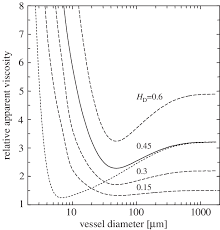The image above represents relative apparent viscosity.

To compute for relative apparent viscosity, two essential parameters are needed and these parameters are Viscosity of Non-Newtonian Fluid (ηa) and Viscosity of Continuous Phase (ηc).

The formula for calculating relative apparent viscosity:

ηra = ηa / ηc

Where:

ηra = Relative Apparent Viscosity
ηa = Viscosity of Non-Newtonian Fluid
ηc = Viscosity of Continuous Phase

Let’s solve an example;
Find the relative apparent viscosity when the viscosity of non-newtonian fluids is 44 and the viscosity of continuous phase is 11.

This implies that;

ηa = Viscosity of Non-Newtonian Fluid = 44
ηc = Viscosity of Continuous Phase = 11

ηra = ηa / ηc
ηra = 44 / 11
ηra = 4

Therefore, the relative apparent viscosity is 4.

Calculating for Viscosity of Non-Newtonian Fluids when the Relative Apparent Viscosity and the Viscosity of Continuous Phase is Given.

ηa = ηra x ηc

Where;

ηa = Viscosity of Non-Newtonian Fluid
ηra = Relative Apparent Viscosity
ηc = Viscosity of Continuous Phase

Let’s solve an example;
Find the viscosity of non-newtonian fluid when the relative apparent viscosity is 16 and the viscosity of continuous phase is 8.

This implies that;

ηra = Relative Apparent Viscosity = 16
ηc = Viscosity of Continuous Phase = 8

ηa = ηra x ηc
ηa = 16 x 8
ηa = 128

Therefore, the viscosity of non-newtonian fluid is 128.

Calculating for the Viscosity of Continuous Phase when the Relative Apparent Viscosity and the Viscosity of Non-Newtonian Phase is Given.

ηc = ηa / ηra

Where;

ηc = Viscosity of Continuous Phase
ηra = Relative Apparent Viscosity
ηa = Viscosity of Non-Newtonian Fluid

Let’s solve an example;
Find the viscosity of continuous phase when the relative apparent viscosity is 14 and the viscosity of non-newtonian fluid is 48.

This implies that;

ηra = Relative Apparent Viscosity = 14
ηa = Viscosity of Non-Newtonian Fluid = 48

ηc = ηa / ηra
ηc = 48 / 14
ηc = 3.428

Therefore, the viscosity of continuous phase is 3.428.

Nickzom Calculator – The Calculator Encyclopedia is capable of calculating the relative apparent viscosity.

To get the answer and workings of the relative apparent viscosity using the Nickzom Calculator – The Calculator Encyclopedia. First, you need to obtain the app.

You can get this app via any of these means:

To get access to the professional version via web, you need to register and subscribe for NGN 2,000 per annum to have utter access to all functionalities.
You can also try the demo version via https://www.nickzom.org/calculator

Apple (Paid) – https://itunes.apple.com/us/app/nickzom-calculator/id1331162702?mt=8
Once, you have obtained the calculator encyclopedia app, proceed to the Calculator Map, then click on Materials and Metallurgical under Engineering.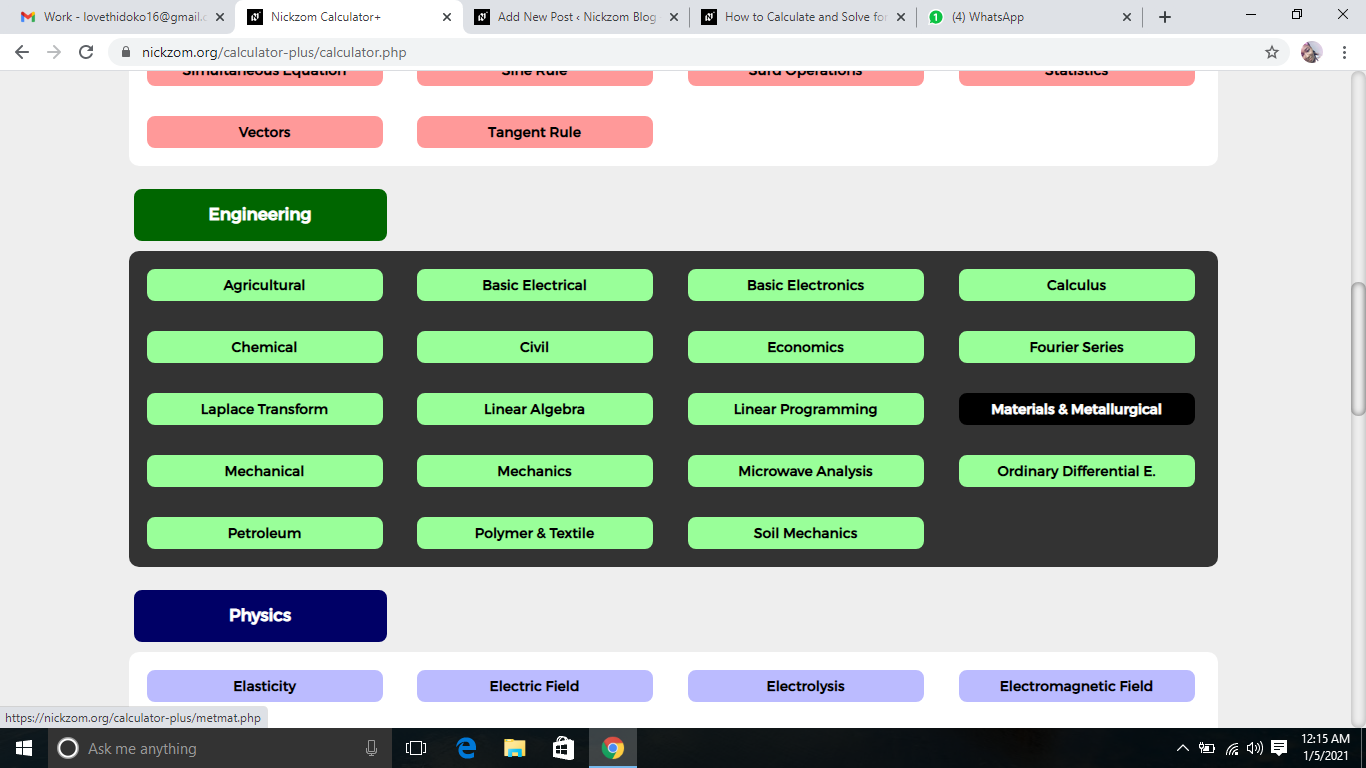Now, Click on Rheology under Materials and Metallurgical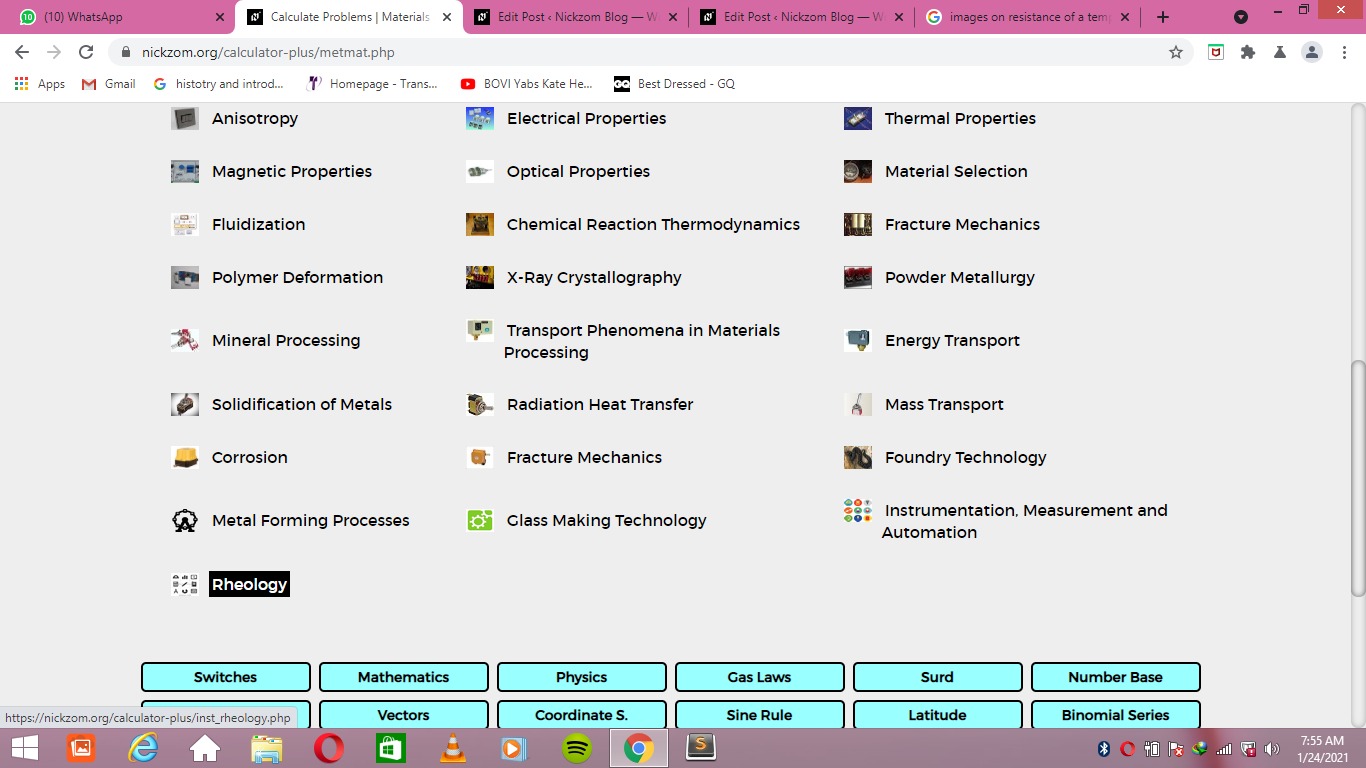Now, Click on Relative Apparent Viscosity under Rheology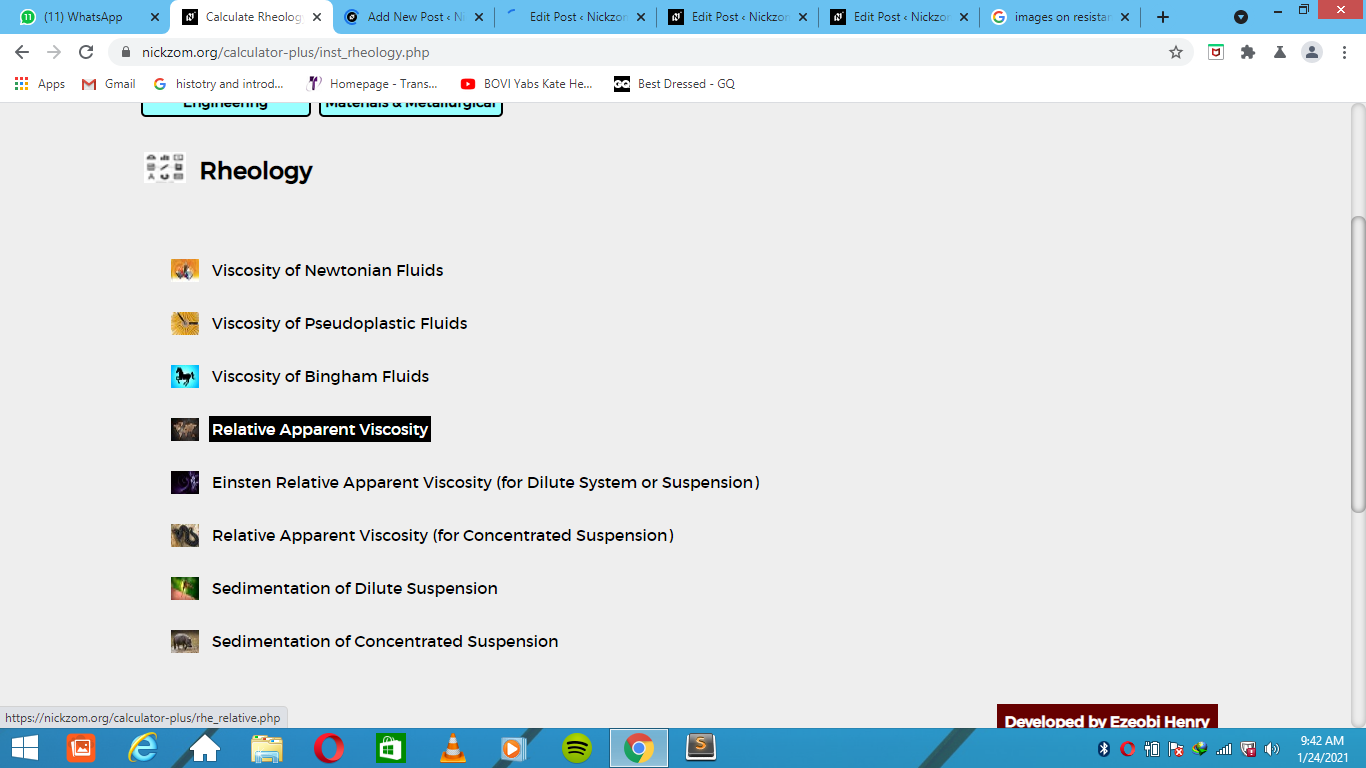The screenshot below displays the page or activity to enter your values, to get the answer for the relative apparent viscosity according to the respective parameters which is the Viscosity of Non-Newtonian Fluid (ηa) and Viscosity of Continuous Phase (ηc).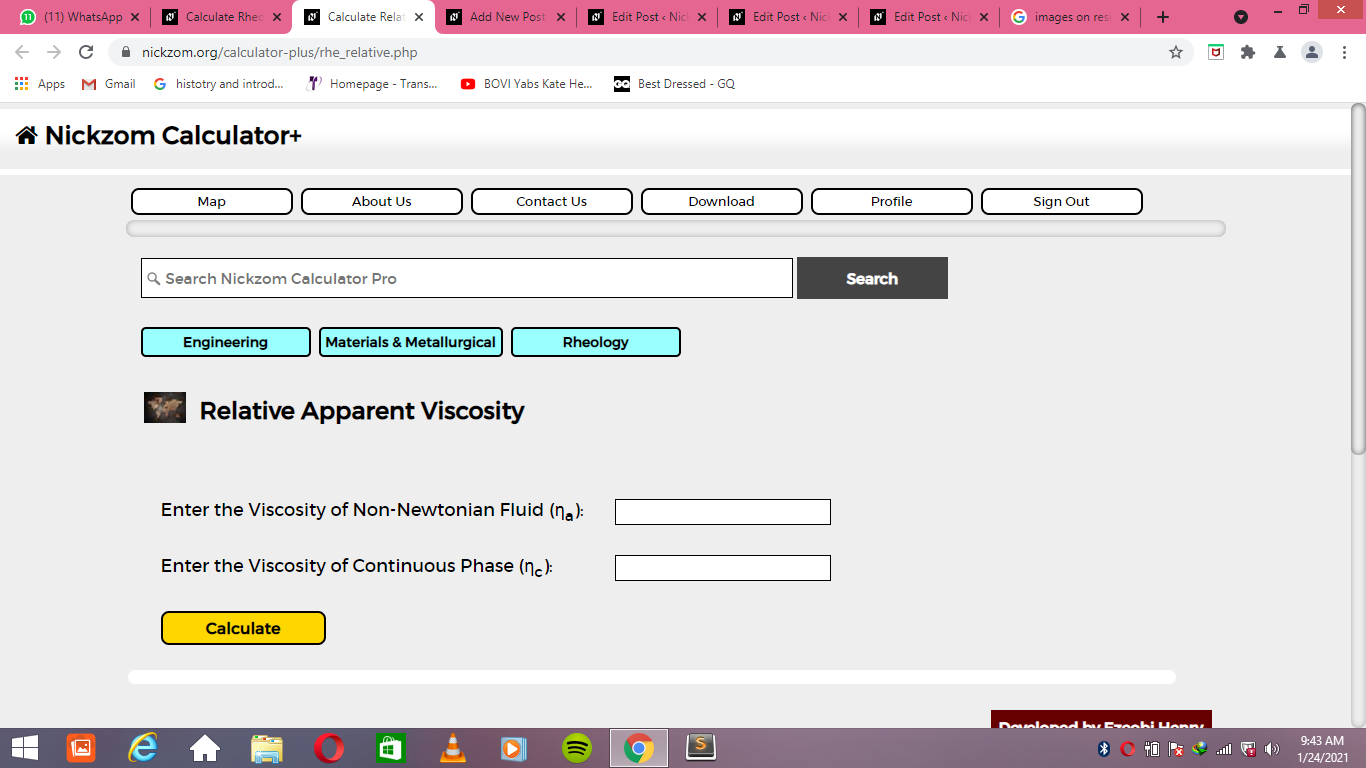Now, enter the values appropriately and accordingly for the parameters as required by the Viscosity of Non-Newtonian Fluid (ηa) is 44 and Viscosity of Continuous Phase (ηc) is 11.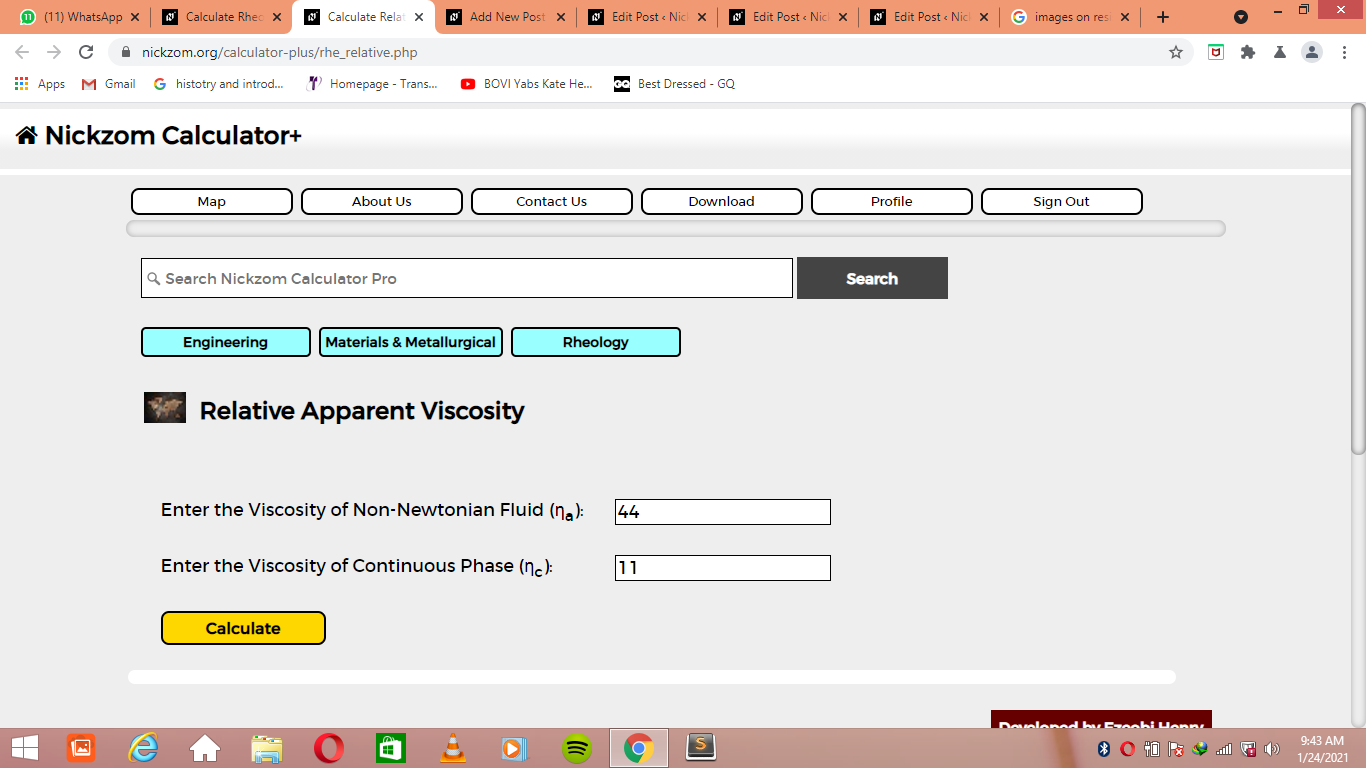Finally, Click on Calculate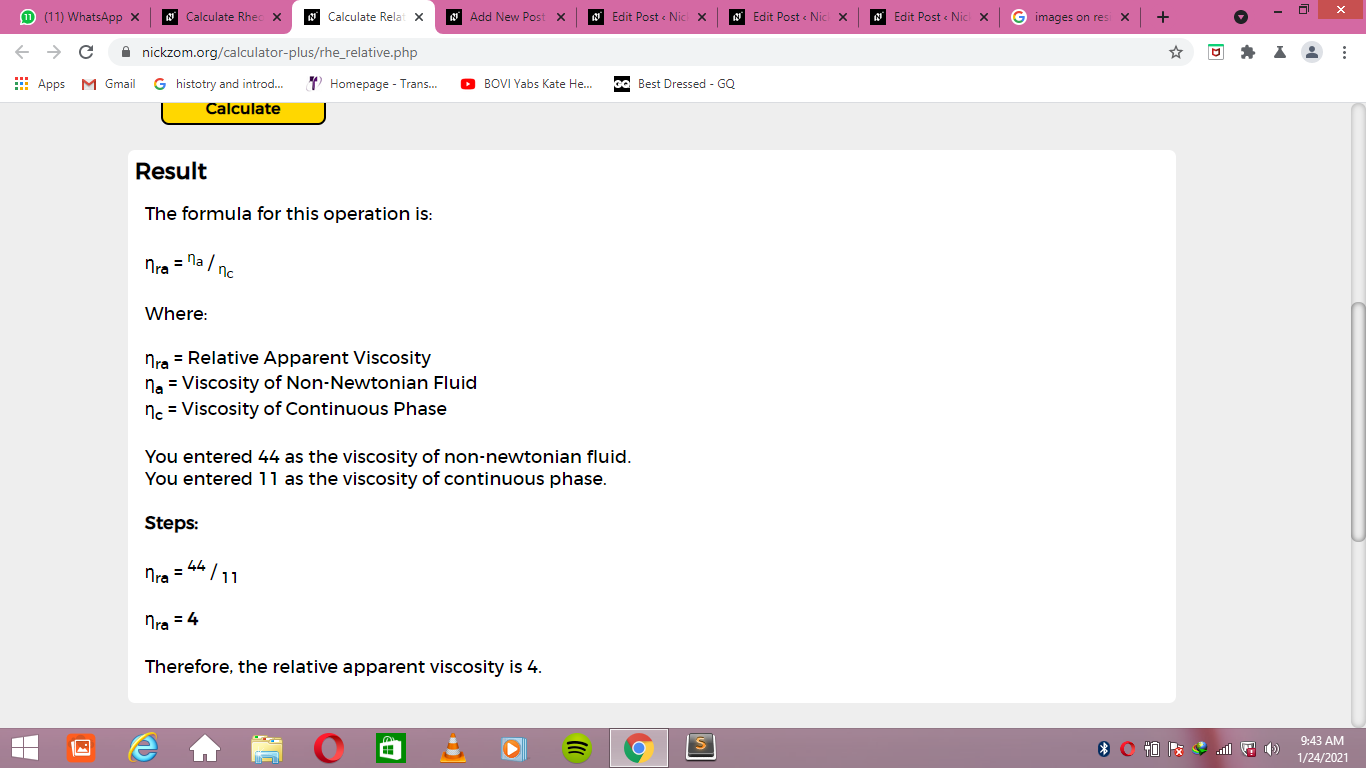As you can see from the screenshot above, Nickzom Calculator– The Calculator Encyclopedia solves for the relative apparent viscosity and presents the formula, workings and steps too.